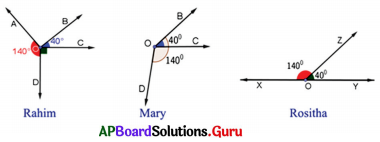# AP Board 7th Class Maths Solutions Chapter 4 Lines and Angles Exercise 4.2

SCERT AP 7th Class Maths Solutions Pdf Chapter 4 Lines and Angles Ex 4.2 Textbook Exercise Questions and Answers.

## AP State Syllabus 7th Class Maths Solutions 4th Lesson Lines and Angles Exercise 4.2

Question 1.
Observe the given figure and write any 2 linear pairs of angles.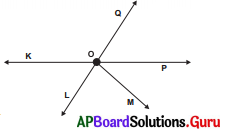Linear pair of angles :
∠KOQ, ∠QOP
∠KOQ, ∠KOL
∠KOL, ∠LOP
∠QOP, ∠POL
∠KOM, ∠MOP
∠QOM, ∠MOL

Question 2.
Draw a pair of adjacent angles which are complementary to each other.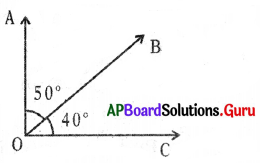In the figure ∠AOB, ∠BOC are adjacent angles and their sum is complementary.
∴ ∠AOB + ∠BOC = 50° + 40° = 90°

Question 3.
Draw a pair of adjacent angles which are supplementary to each other.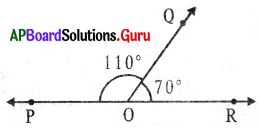In the figure ∠POQ, ∠OOR are linear pair and adjacent angles and their sum is supplementary.
∴ ∠POQ + ∠OOR = 110° + 70° = 180°

Question 4.
Fan, Bullock cart wheel, Clock, etc.Question 5.
Observe the figure and write the adjacent angles.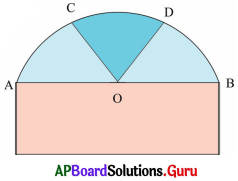In the given figure, adjacent angles are
∠AOC, ∠COB
∠AOC, ∠COD
∠COD, ∠DOB
∠AOD, ∠DOB

Question 6.
Is it possible for the following pair of angles to form a linear pair? If yes, draw them. If not, give reason.
(i) 120°, 60°
Yes.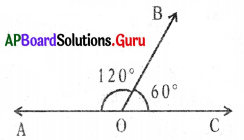120° + 60° = 180°
120°, 60° (Linear pair)

(ii) 98°, 102°
No, their sum is not equal to 180°
98° + 102° = 200° > 180°
So, they are not 1irear pair.

Question 7.
Draw the following angles as linear pair. Write the straight line and common arm.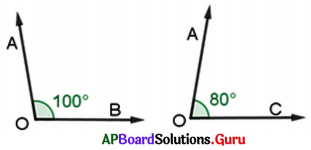∠BOA + ∠AOC = 180°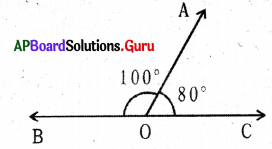∴ ∠BOA and ∠AOC are linear pair.
BC is a straight line and OA is an arm.

Question 8.
In the given figure, AB is a straight line. O is a point on AB . Find the value of x.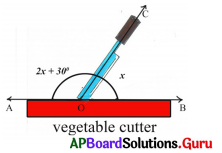In the given figure $$\overrightarrow{\mathrm{AB}}$$ is a straight line.
∠AOC + ∠COB = 180°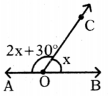⇒ 2x + 30° + x = 180°
⇒ 3x + 30°= 180°
⇒ 3x + 30° – 30°= 180°
⇒ 3x = 150°
⇒ $$\frac{3 x}{3}=\frac{150^{\circ}}{3}$$
⇒ x = 50Question 9.
Teacher asked students to check whether 40° and 140° form a linear pair or not by drawing angles. The students have drawn as follows. Who will get correct answer?